## A Quick Word on Julian Assange

Either you are horrified by the persecution of Julian Assange or, like Lisa Millar and Patricia Karvelas and Peter Greste and Michael “Gold Star” Rowland, you support the fascistic, war-mongering motherfuckers out to get him. There is no middle ground.

## Update (31/07/19)

ABC’S Four Corners has just aired a 2-part program on Julian Assange. It is well-made, interesting and, in keeping with modern journalistic style, entirely without self-awareness and entirely off the fucking point.

## WitCH 17: Compounding Our Problems

The WitCHfest is coming to an end. Our final WitCH is, once again, from Cambridge’s Specialist Mathematics 3 & 4 (2019). The section establishes the compound angle formulas, the first proof of which is our WitCH.## Update (25/08/19)

Similar to our parallel WitCH, it is difficult to know whether to focus on specific clunkiness or intrinsic absurdity, but we’ll first get the clunkiness out of the way:

• John comments that using x and y for angles within the unit circle is irksome. It is more accurately described as idiotic.
• The 2π*k is unnecessary and distracting, since the only possible values of k are 0 and -1. Moreover, by symmetry it is sufficient  to prove the identity for x > y, and so one can simply assume that x = y + α.
• The spacing for the arguments of cos and sin are very strange, making the vector equations difficult to read.
• The angle θ is confusing, and is not incorporated in the proof in any meaningful manner.
• Having two cases is ugly and confusing and was easily avoidable by an(other) appeal to trig symmetry.

In summary, the proof could have been much more elegant and readable if the writers had bothered to make the effort, and in particular by making the initial assumption that yxy + π, relegating other cases to trig symmetry.

Now, to the general absurdity.

It is difficult for a textbook writer (or a teacher) to know what to do about mathematical proofs. Given that the VCAA doesn’t give a shit about proof, the natural temptation is to pay lip service or less to mathematical rigour. Why include a proof that almost no one will read? Commenters on this blog are better placed to answer that question, but our opinion is that there is still a place for such proofs in school texts, even if only for the very few students who will appreciate them.

The marginalisation of proof, however, means that a writer (or teacher) must have a compelling reason for including a proof, and for the manner in which that proof is presented. (This is also true in universities where, all too often, slovenly lecturers present  incomprehensible crap as if it is deep truth.) Which brings us to the above proof. Specialist 34 students should have already seen a proof of the compound angle formulas in Specialist 12, and there are much nicer proofs than that above (see below). So, what is the purpose of the above proof?

As RF notes, the writers are evidently trying to demonstrate the power of the students’ new toy, the dot product. It is a poor choice, however, and the writers in any case have made a mess of the demonstration. Whatever elegance the dot product might have offered has been obliterated by the ham-fisted approach. Cambridge’s proof can do nothing but convince students that “proof” is an incomprehensible and pointless ritual. As such, the inclusion of the proof is worse than having included no proof at all.

This is doubly shameful, since there is no shortage of very nice proofs of the compound angle formulas. Indeed, the proof in Cambridge’s Specialist 12 text, though not that pretty, is standard and is to be preferred. But the Wikipedia proof is much more elegant. And here’s a lovely proof of the formula for sin(A + B) from Roger Nelson’s Proof Without Words: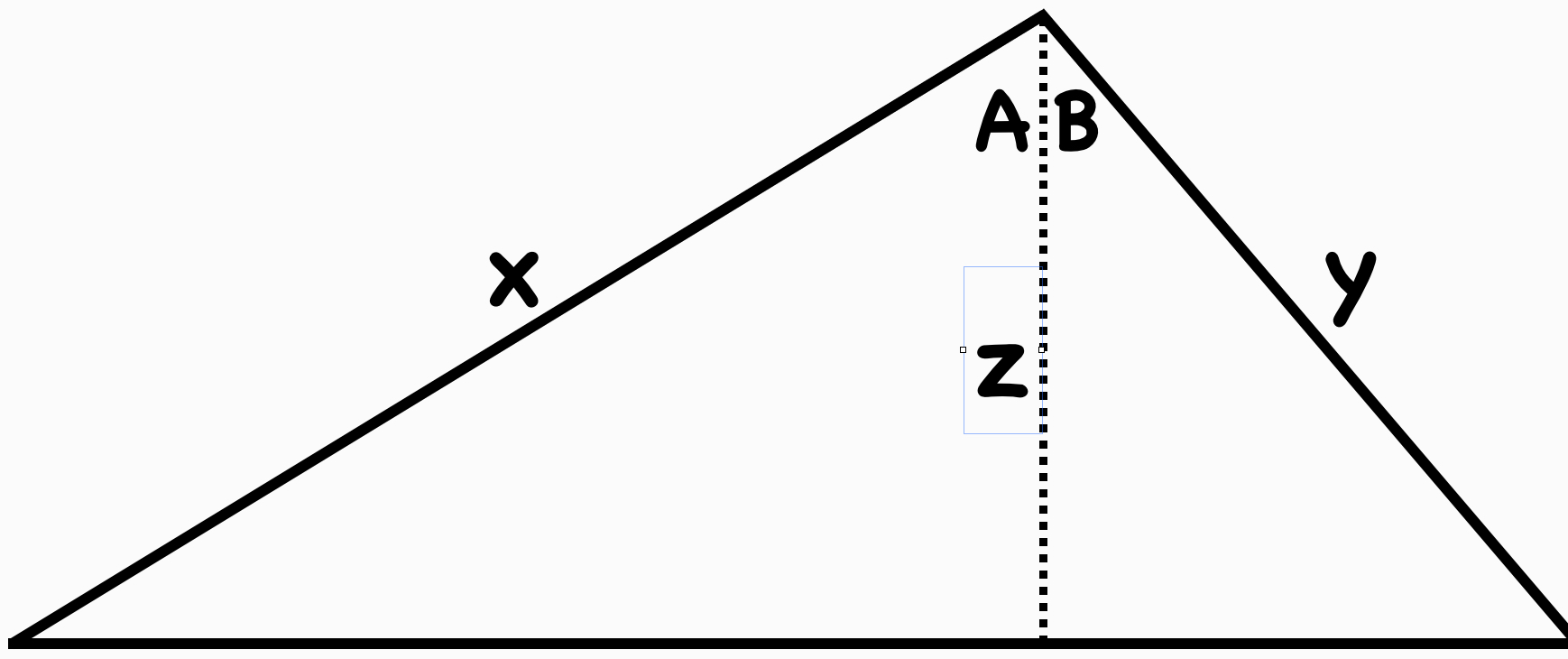To make the proof work, just note that

x cos(A) = z = y cos(B)

Now write the area of the big triangle in two different ways, and you’re done. A truly memorable proof. That is, a proof with a purpose.

## WitCH 16: The Root of Our Problem

This WitCH comes from one of our favourites, the Complex Numbers chapter from Cambridge’s Specialist Mathematics 3 & 4 (2019). It is not as deep or as beWitCHing as other aspects of the chapter. But, it’s still an impressive WitCH.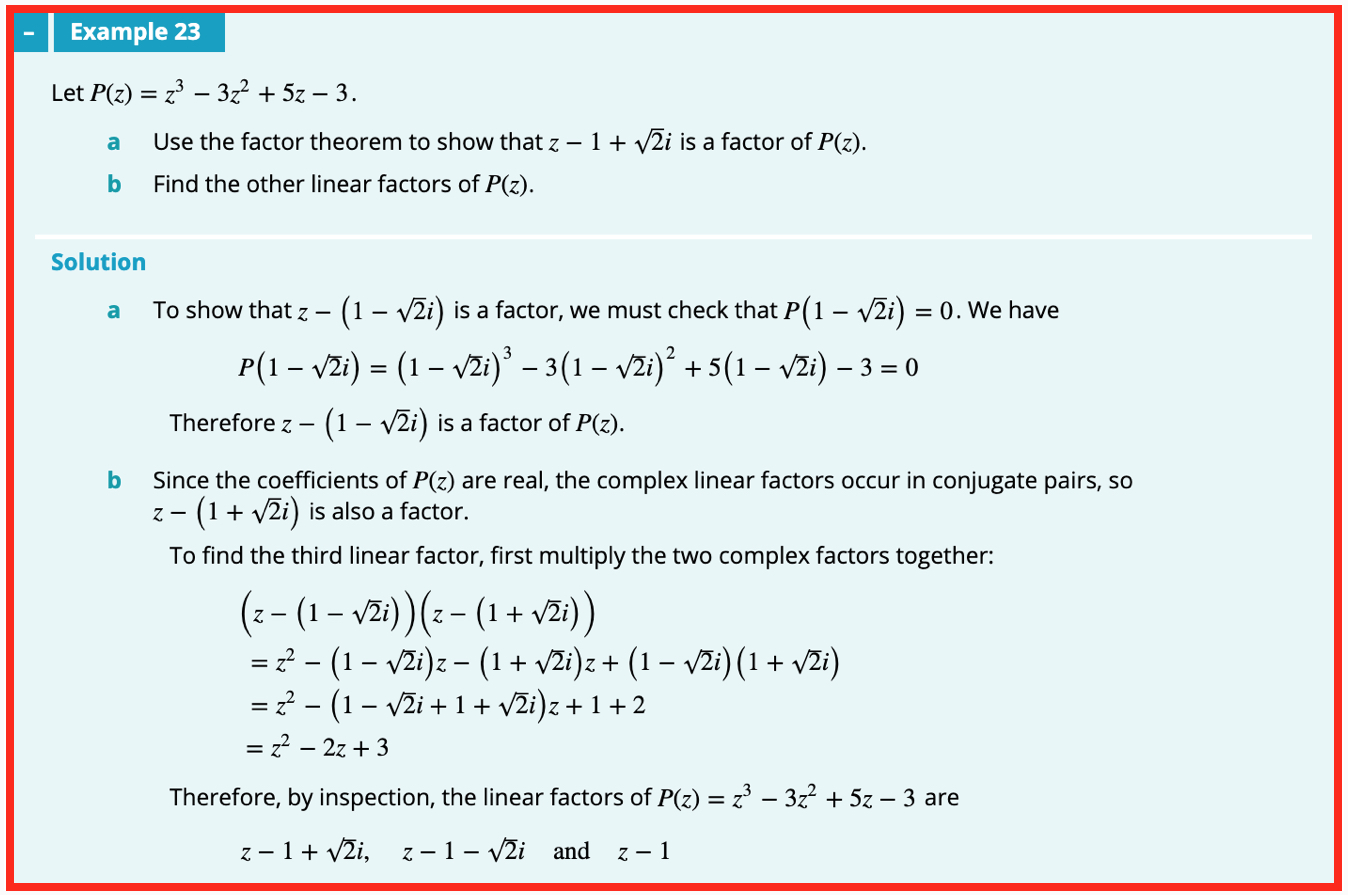## Update (11/08/19)

I guess if you’re gonna suggest a painful, ass-backwards method to solve a problem, you may as well fake the solution:

• Checking directly that P(1 – i√2) = 0 involves expanding a cubic, and more, which the text does in one single magic line.
• The painful multiplication of the products for part b is much more naturally and easily done as a difference of two squares: (z – 1 – i√2)(z – 1 + i√2) = (z – 1)2 + 2, etc.
• After all that the third factor, z – 1, is determined “by inspection”? Inspection of what?

AS RF notes, it is much easier to spot that z = 1 solves the cubic. Then some easy factoring (without long division …) gives P = (z – 1)(z2 – 2z + 3). Completing the square then leads to the linear factors, answering both parts of the question in the reverse, and natural, order.

Alternatively, as John notes, the difference of two squares calculation shows that if z – 1 + i√2 is a factor of P then so is the quadratic z^2 – 2z + 3. That this is so can then be checked (without long division …), giving P = (z – 1)(z^2 – 2z + 3), and so on, as before.

## WitCH 15: Principled Objection

OK, playtime is over. This one, like the still unresolved WitCH 8, will take some work. It comes from Cambridge’s Mathematical Methods 3 & 4 (2019). It is the introduction to “When is a function differentiable?”, the final section of the chapter “Differentiation”.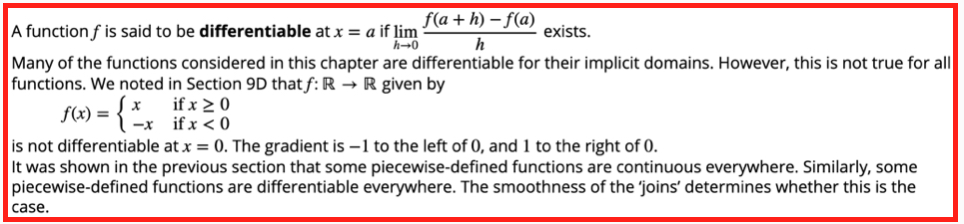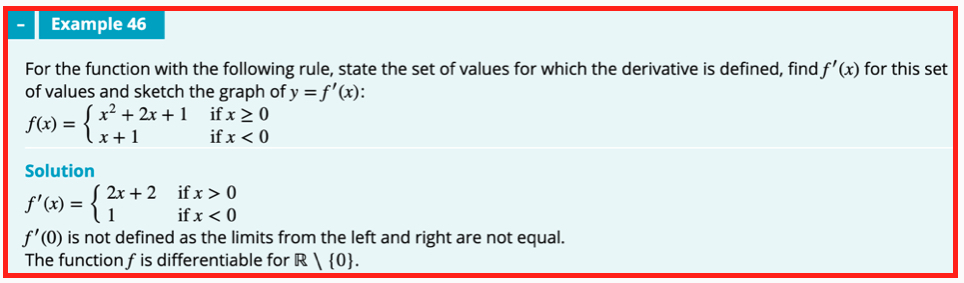## Update (12/08/19)

We wrote about this nonsense seven long years ago, and we’ll presumably be writing about it seven years from now. Nonetheless, here we go.

The first thing to say is that the text is wrong. To the extent that there is a discernible method, that method is fundamentally invalid. Indeed, this is just about the first nonsense whacked out of first year uni students.

The second thing to say is that the text is worse than wrong. The discussion is clouded in gratuitous mystery, with the long-delayed discussion of “differentiability” presented as some deep concept, rather than simply as a grammatical form. If a function has a derivative then it is differentiable. That’s it.

Now to the details.

The text’s “first principles” definition of differentiability is correct and then, immediately, things go off the rails. Why is the function f(x) = |x| (which is written in idiotic Methods style) not differentiable at 0? The wording is muddy, but example 46 makes clear the argument: f’(x) = -1 for x < 0 and f’(x) = 1 for x > 0, and these derivatives don’t match. This argument is unjustified, fundamentally distinct from first principles, and it can easily lead to error. (Amusingly, the text’s earlier, “informal” discussion of f(x) = |x| is exactly what is required.)

The limit definition of the derivative f’(a) requires looking precisely at a, at the gradient [f(a+h) – f(a)]/h as h → 0. Instead, the text, with varying degrees of explicitness and correctness, considers the limit of f’(x) near a, as x → a. This second limit is fundamentally, conceptually different and it is not guaranteed to be equal.

The standard example to illustrate the issue is the function f(x) = x2sin(1/x) (for x≠ 0 and with f(0) = 0). It is easy to to check that f’(x) oscillates wildly near 0, and thus f’(x) has no limit as x → 0. Nonetheless, a first principles argument shows that f’(0) = 0.

It is true that if a function f is continuous at a, and if f’(x) has a limit L as x → a, then also f’(a) = L. With some work, this non-obvious truth (requiring the mean value theorem) can be used to clarify and to repair the text’s argument. But this does not negate the conceptual distinction between the required first principles limit and the text’s invalid replacement.

Now, to the examples.

Example 45 is just wrong, even on the text’s own ridiculous terms. If a function has a nice polynomial definition for x ≥ 0, it does not follow that one gets f’(0) for free. One cannot possibly know whether f’(x) exists without considering x on both sides of 0. As such, the “In particular” of example 46 is complete nonsense. Further, there is the sotto voce claim but no argument that (and no illustrative graph indicating) the function f is continuous; this is required for any argument along the text’s lines.

Example 46 is wrong in the fundamental wrong-limit manner described above. it is also unexplained why the magical method to obtain f’(0) in example 45 does not also work for example 46.

Example 47 has a “solution” that is wrong, once again for the wrong-limit reason, but an “explanation” that is correct. As discussed with Damo in the comments, this “vertical tangent” example would probably be better placed in a later section, but it is the best of a very bad lot.

And that’s it. We’ll be back in another seven years or so.

## WitCH 14: Stretching the Truth

The easy WitCH below comes courtesy of the Evil Mathologer. It is a worked example from Cambridge’s Essential Mathematics Year 9 (2019), in a section introducing parabolic graphs.## Update

The problem, as commenters have indicated below, is that there is no parabola with the indicated turning point and intercepts. Normally, we’d write this off as a funny but meaningless error. But, coming at the very beginning of the introduction to the parabola, it most definitely qualifies as crap.

## WitCH 13: Here for the Ratio

The WitCH below is courtesy of a clever Year 11 student. It is a worked example from Jacaranda’s Maths Quest 11 Specialist Mathematics (2019):Update (11/08/19)

It is ironic that a solution with an entire column of “Think” instructions exhibits so little thought. Who, for example, thinks to “redraw” a diagram by leaving out a critical line, and by making an angle x/2 appear larger than the original x? And it’s downhill from there.

The solution is painfully long, the consequence of an ill-chosen triangle, requiring the preliminary calculation of a non-obvious distance. As Damo indicates, the angle x is easily determined, as in the following diagram: we have tan(x/2) = 1/12, and we’re all but done.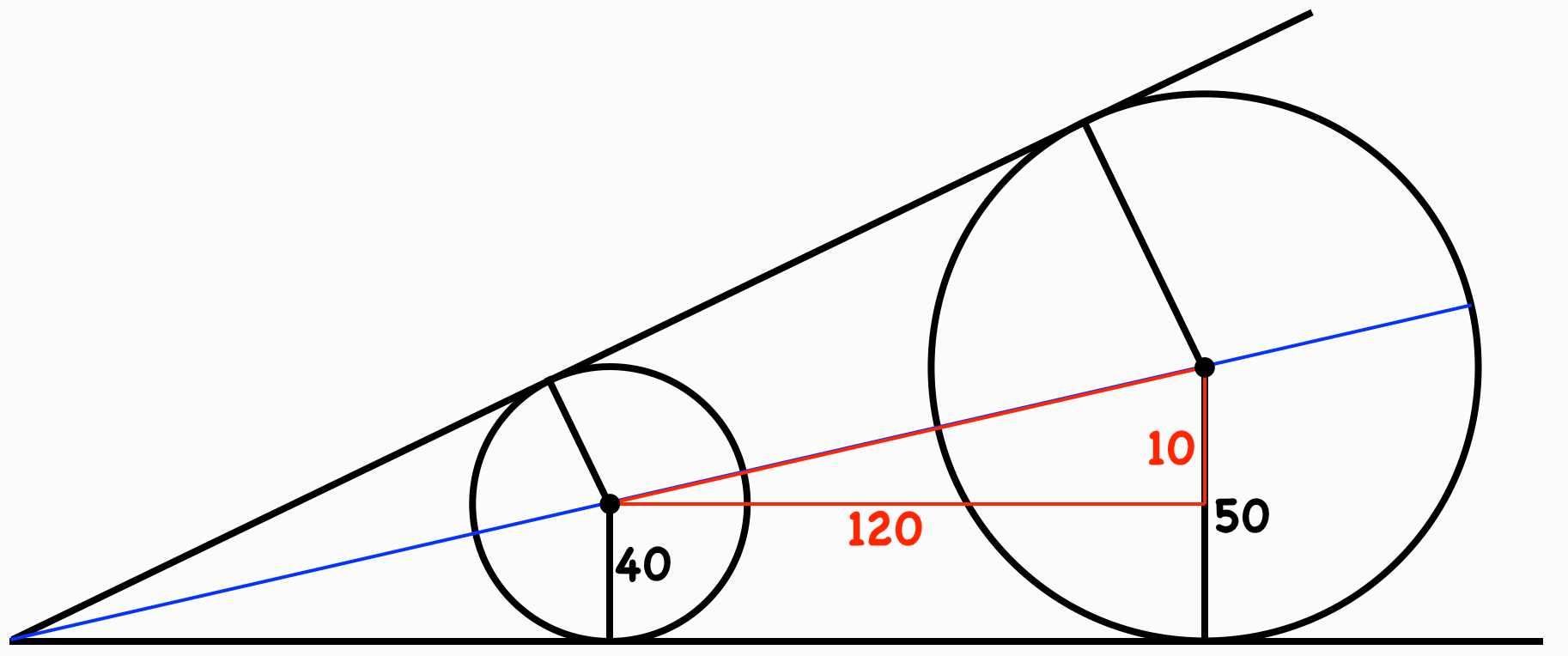(It is not completely obvious that the line through the circle centres makes an angle x/2 with the horizontal, though this follows easily enough from our diagram. The textbook solution, however, contains nothing explicit or implicit to indicate why the angle should be so.)

But there is something more seriously wrong here than the poor illustration of a poorly chosen solution. Consider, for example, Step 5 (!) where, finally, we have a suitable SOHCAHTOA triangle to calculate x/2, and thus x. This simple computation is written out in six tedious lines.

The whole painful six-step solution is written in this unreadable we-think-you’re-an-idiot style. Who does this? Who expects anybody to do this? Who thinks writing out a solution in such excruciating micro-detail helps anyone? Who ever reads it? There is probably no better way to make students hate (what they think is) mathematics than to present it as unforgiving, soulless bookkeeping.

And, finally, as Damo notes, there’s the gratuitous decimals. This poison is endemic in school mathematics, but here it has an extra special anti-charm. When teaching ratios don’t you “think”, maybe, it’s preferable to use ratios?

## PoSWW 7: Power Outage

This PoSWW comes courtesy of our friend Alison. (Alison is the feistiest Christian since Jesus Christ.) The PoSSW was from a worksheet inflicted upon Alison’s Year 8-9 daughter. We don’t know the publisher of the worksheet.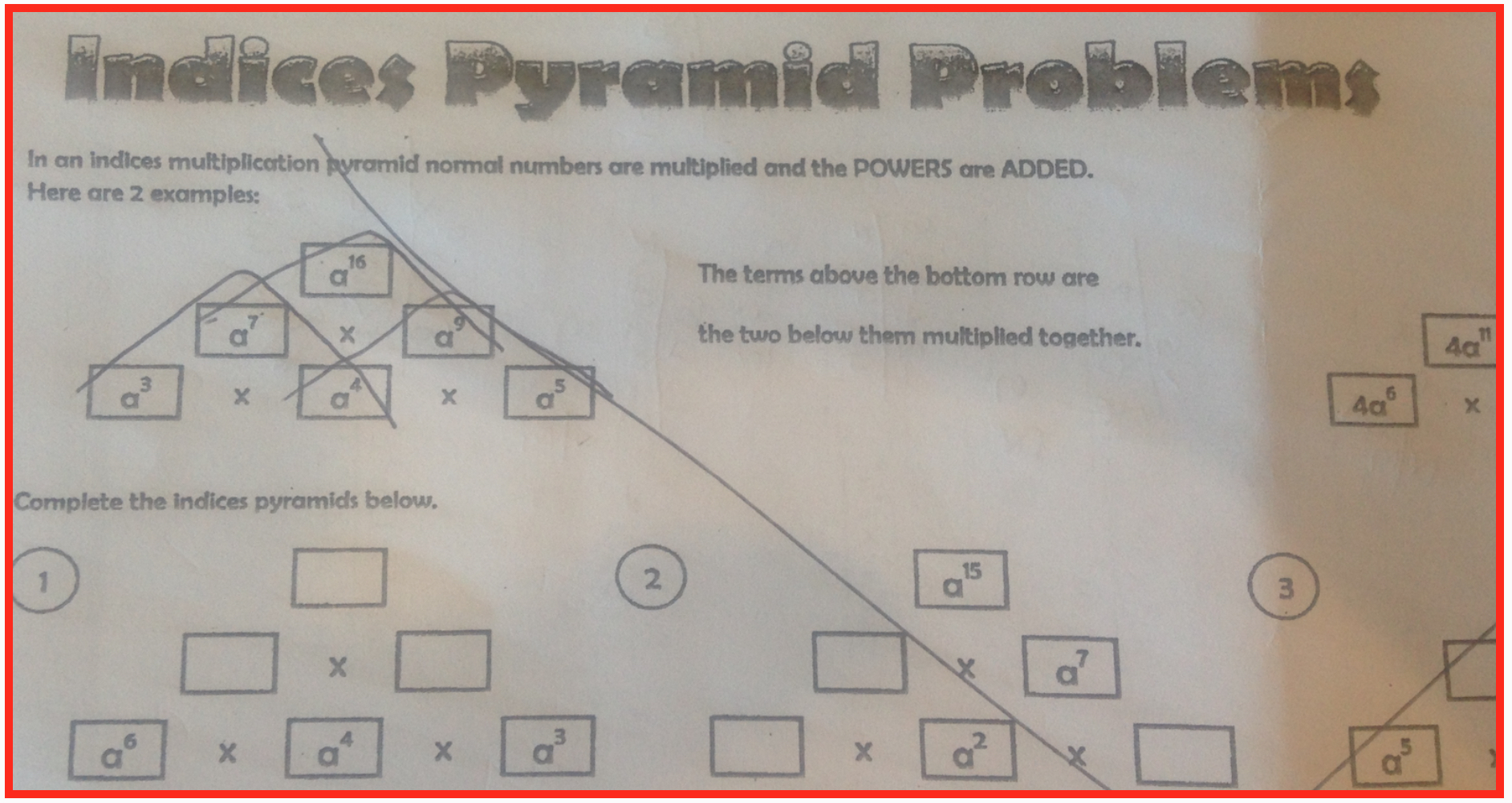## PoSWW 6: Logging Off

The following exercise and, um, solution come from Cambridge’s Mathematical Methods 3 & 4 (2019):## Update

Reflecting on the comments below, it was a mistake to characterise this exercise as a PoSWW; the exercise had a point that we had missed. The point was to reinforce the Magrittesque lunacy inherent in Methods, and the exercise has done so admirably. The fact that the suggested tangents to the pictured graphs are not parallel adds a special Methodsy charm.## WitCH 12: Duplicitous

The following WitCH comes from the VCE 2014 Specialist Exam 1: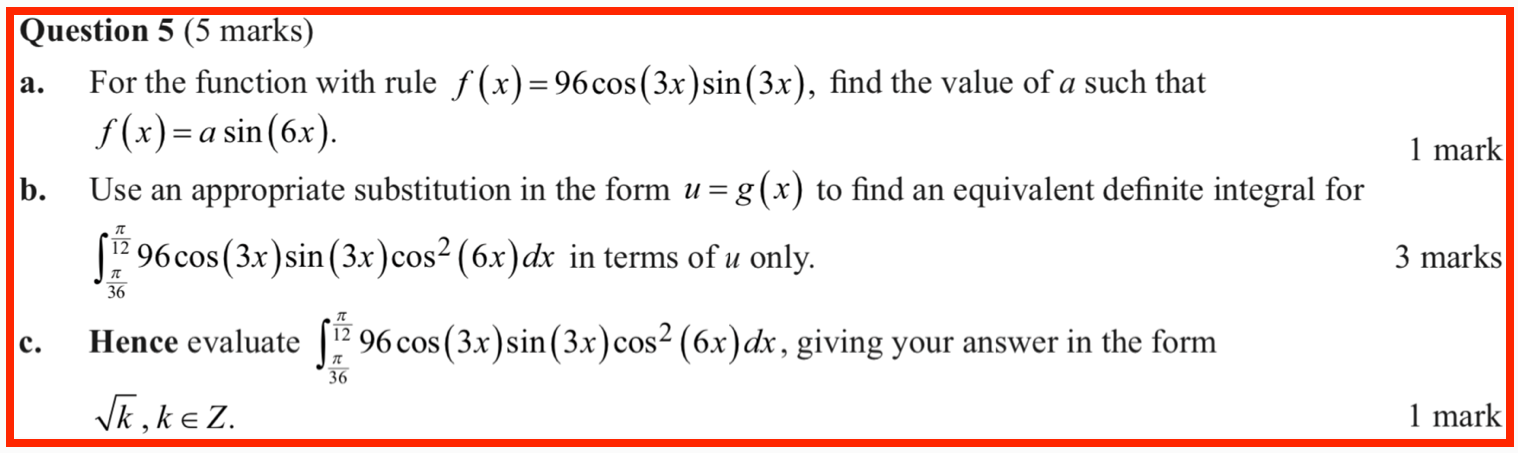The Examiners’ Report indicates that 81%, 48% and 45% of students received full marks for parts (a), (b) and (c), respectively.

## WitCH 11: Impartial

The following WitCH comes from (CAS permitted) 2018 Specialist Mathematics Exam 2: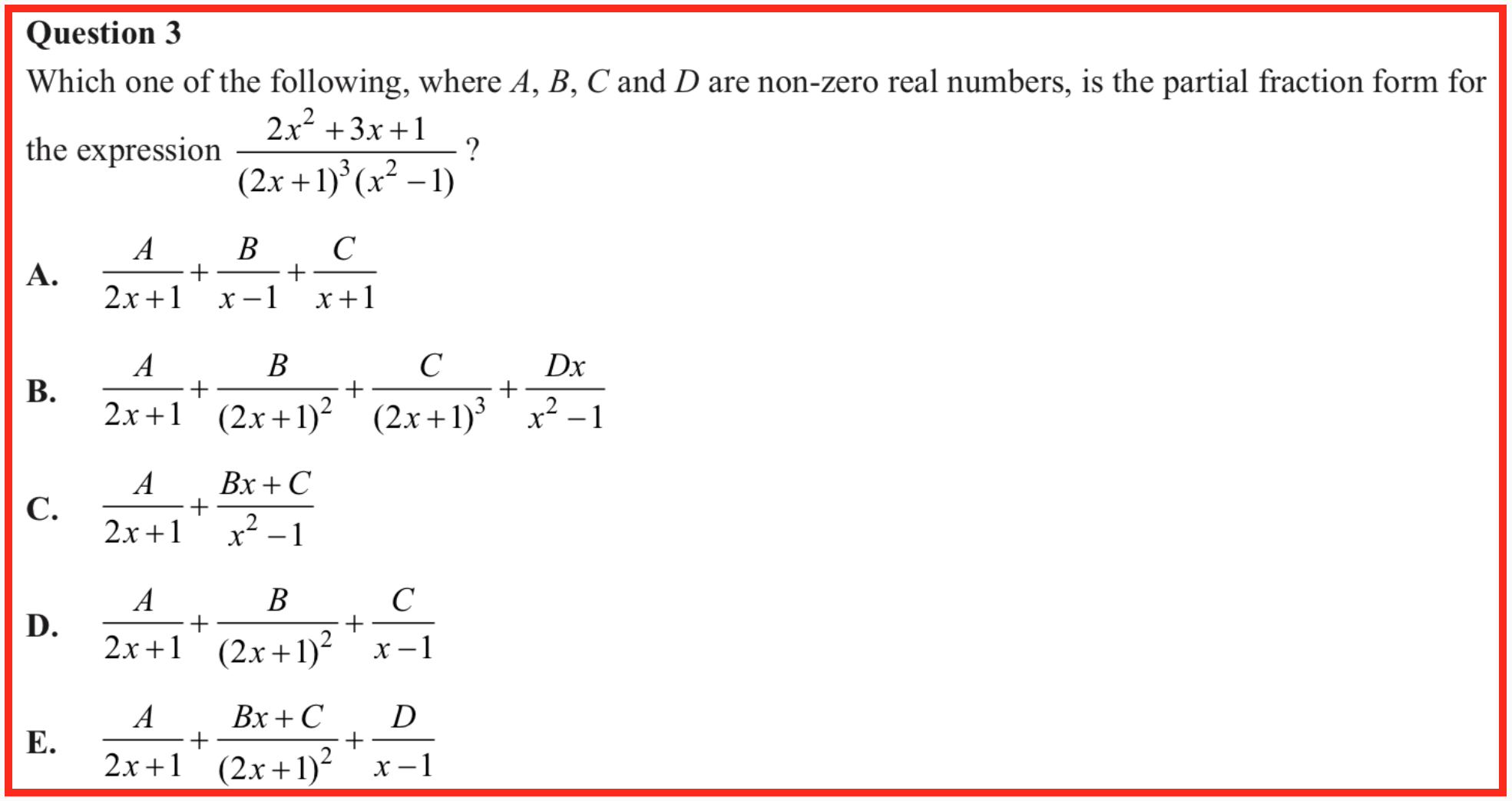The Examiners’ Report indicates that about half of the students gave the intended answer of D, with about a third giving the incorrect answer B. The Report notes:

Option B did not account for common factors and its last term is not irreducible, so should not have Dx in the numerator.

## Update (11/08/19)

The worst kind of exam question is one that rewards mindless button-pushing and actively punishes intelligent consideration. The above question is of the worst kind. It is also pointless, nasty and self-trippingly overcute.

As John points out in the comments, the question can simply be done by pressing CAS buttons. But, alternatively, the question also just appears to require, and to invite, a simple understanding of partial fraction form. Which brings us to the nastiness: the expected partial fraction form is not a listed option.

So, what to make of it? Not surprisingly, many students opted for B, the superficially most plausible answer. A silly mistake, you silly, silly student! You shoulda just listened to your teacher and pushed the fucking buttons.

The trick, of course, is that the numerator factorises, cancelling with the denominator and leading to the intended answer, D. The problem with the trick is that it is antimathematical and wrong:

• As Damo notes, the original rational function is undefined at x = -1, which is lost in the intended answer.
• As Damo also points out, there is no transparent, non-computational way to check that the coefficients in answer D would, as demanded by the question, be non-zero.
• It is not standard or particularly natural to hunt for common factors before breaking into partial fractions. Any such factors will anyway become apparent in the partial fractions.
• To refer to the partial fraction form is actively misleading. Though partial fraction decomposition can be defined so as to be unique, in practice it is usually not helpful to do so, and the VCE Study Design never does so. In particular, if answer B had contained a final numerator of Dx + E then this answer would be valid and, in certain contexts, natural and useful.
• The examiners’ comment on answer B is partly wrong and partly incomprehensible. One can pedantically object to the reducible denominator but if that is the objection then why whine about the Dx in the numerator? And yes, answer B is missing the constant E, which in general is required, and happens to be required for the given rational function. For a specific rational function, however, one might have E = 0. Which brings us back to Damo’s point, that without actually computing the partial fractions there is no way of determining whether answer B is valid.

But of course all that is way, way too much to think about in a speed-test exam. Much better to just listen to your teacher and push the fucking buttons.